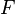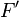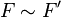Similarity (geometry) facts for kids

Kids Encyclopedia Facts

Similarity is an idea in geometry. It means that two polygons, line segments, or other figures can become the same via resizing. Similar objects do not need to have the same size. Two shapes are similar if their angles have the same measure and their sides are proportional. Two circles, squares, or line segments are always similar. If figure$F$ is similar to figure$F'$, then we write$F \sim F'$.

When it comes to similarity, triangles are special. This is because triangles can be similar if only their angles are equal, or only their sides are proportional. All other polygons must meet both of the conditions.

Similarity is very similar to congruence. Congruent shapes have the same sides and angles. Because of that, two shapes are congruent to each other if one can become another through rotating, reflecting or moving only. In fact, all shapes that are congruent to each other are also similar, but not vice versa.

Related pagesSimilarity (geometry) Facts for Kids. Kiddle Encyclopedia.